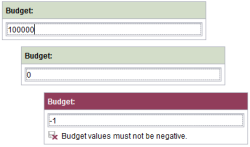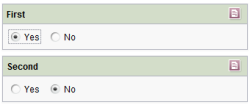## VALUE property

### Using the value of an input component

FirstSpirit input components can take a value and save it persistently. The VALUE property is used to read out the value from an input component or write a value to an input component:

1. Within the value determination: using the VALUE property, the value from an input component can be read out in a form and evaluated using a dynamic form. This makes it possible, for instance, to define rules that first read out the value placed in an input component and then check the value's particular properties, such as the number of characters (using the LENGTH attribute) or the structure via a regular expression (see <MATCHES/>).
2. Within a handling instruction: the VALUE property can also be used to place values in input components. This makes it possible, for instance, to use a dynamic form to first read out a value from an input component, process it further (see <ADD/>) and then place it in a different input component of the form.

• returns the value of the specified input component (when used within the value determination) or
• sets the previously determined value in the specified input component (when used within the handling instruction)

If the property is set within the value determination area, to define a complete condition for value-dependent validation, such as:

• “Is the value of input component A greater than the value in input component B” or
• “Does the value in the input component correspond to the following regular expression”,

additional information may be needed, such as a comparison value (depending on the input component); for example:

• a constant text comparison value (e.g. <TEXT>EN</TEXT>) or
• a constant, numeric comparison value (for example, <NUMBER>9</NUMBER>) or
• a variable comparison value, e.g. the value from a different input component (<PROPERTY source='gadget' name='VALUE'/> )

and an operator, such as:

In this case, the expression will return a Boolean value.

### Exception: setting dynamic values using a database query

When setting values within the handling instruction of a rule, exceptions are when the selection quantity of a

This is carried out by using a database query via the CMS_INCLUDE_OPTIONS tag (in the input component form definition).

For this purpose, a value is obtained within value determination area (using the VALUE property) first, which is then to be used for the database query. This can be a constant value or the value of another input component. This value is then to be implemented as a filter parameter for the database query. The following expression (in dot notation) is used for this purpose within the handling instruction:
<PROPERTY source="combobox" name="query.identifier"/>

The <PROPERTY/> tag is used to specify the “combobox” input component to be populated (via the source attribute) and the valid identifier for the database query to be used with the “query” prefix (via the name attribute). The value from the value determination section is then used as the filter parameter (FILTERPARAM) in the corresponding database query (check for type consistency). The selection quantity of the CMS_INPUT_COMBOBOX will now be populated dynamically via a database query (defined for this component in the form), where the value quantity is filtered using the parameter determined in the rule.

For an example, see: Dish / supplier and Determining a substitute

## Examples

### Example 1) Preventing input of negative valuesThe following form contains a “st_budget” input component (of the type CMS_INPUT_NUMBER). A dynamic form is used to ensure that the editor cannot input any negative numerical values in the input field.

In addition, within the <WITH/> section, the value of the input component is retrieved and compared against a numerical constant (0 in this case). The result of this “less-than” check is a Boolean value which is then linked with a validation of the input component in the <DO/> section. As long as the condition (“Value is not less than 0”) is not fulfilled, the <VALIDATION/> section of the rule is run and a correction notice appears. The SAVE/> attribute also prevent saving of the form.

`...<RULE>    <WITH>        <NOT>            <LESS_THAN>                <PROPERTY source="st_budget" name="value"/>                <NUMBER>0</NUMBER>            </LESS_THAN>        </NOT>    </WITH>    <DO>        <VALIDATION scope="SAVE">            <PROPERTY source="st_budget" name="VALID"/>            <MESSAGE lang="*" text="Budget values must not be negative."/>            <MESSAGE lang="DE" text="Budgetkosten dürfen nicht negativ sein"/>        </VALIDATION>    </DO></RULE>...`

### Example 2) Setting a value in an input component (depending on the second value)The following form contains two input components: “first” and “second” (of the CMS_INPUT_TOGGLE type). A dynamic form is used to ensure that when the editor changes the value of the first input component, the value of the second input component is adapted automatically. If the editor changes the value of the first component from “Yes” to “No”, the value of the second component will therefore also switch automatically from “No” to “Yes” (and vice versa).

In addition, the value of the first input component is retrieved within the <IF/> section. Only after the precondition (value of the first input component is “Yes”) is fulfilled will the subsequent sections of the rule be carried out. Within the <WITH/> section, the <FALSE/> constant is set in order to apply the negative value in the <DO/> section to the second input component.
first = = yes sets second = = no

To check the second condition (value of the first input component is “No”), the precondition must be negated. In this case, the precondition is fulfilled and the subsequent rule section is executed. Within the <WITH/> section, the <TRUE/> constant is set in order to apply this positive value in the <DO/> section to the second input component.
first = = no sets second = = yes

`...<!-- When "first" is set to "Yes", then set "second" to "No" --><RULE> <IF> <EQUAL> <PROPERTY name="VALUE" source="First"/> <TRUE/> </EQUAL> </IF> <WITH> <FALSE/> </WITH> <DO> <PROPERTY name="VALUE" source="Second"/> </DO></RULE><!-- When "first" is set to "No", then set "second" to "Yes" --><RULE> <IF> <EQUAL> <PROPERTY name="VALUE" source="First"/> <FALSE/> </EQUAL> </IF> <WITH> <TRUE/> </WITH> <DO> <PROPERTY name="VALUE" source="Second"/> </DO></RULE>´...`

### Example 4) Determining substitutes (rules and database queries)

For an explanation, see Exception: setting dynamic values using a database query.

The following form contains:

• two components of the type CMS_INPUT_TEXT for entering an employee's first name (“cs_firstname”) and last name (“cs_lastname”),
• the combo box “cs_department” for entering a department, and
• the combo box “cs_substitute” for selecting a substitute for the employee. The combo box item selection quantity is populated dynamically using a database query and provides the names of the available employees from the corresponding department (minus the employee for whom the substitute is determined) for selection.

Template (form) - combo box with table template information and query tag:

The CMS_INCLUDE_OPTIONS tag is dynamically populated with the value quantity of the combo box from the “employee” (database) table (<TABLE/>). In addition, use of the database query (“possible_substitutes_query”) is defined by the <QUERY/> tag in the form in order to limit the quantity of available datasets.

`...<CMS_INPUT_COMBOBOX name="cs_substitute" useLanguages="no">    <CMS_INCLUDE_OPTIONS type="database">        <LABELS>            <LABEL lang="*">#item.last_name + ", " + #item.first_name</LABEL>        </LABELS>        <QUERY name="possible_substitutes_query"/>        <TABLE>employee</TABLE>    </CMS_INCLUDE_OPTIONS>    <LANGINFOS>        <LANGINFO lang="*" label="Substitute"/>        <LANGINFO lang="DE" label="Vertretung"/>    </LANGINFOS></CMS_INPUT_COMBOBOX>...`

Query (“possible_substitutes_query”):

The database query defined in the form filters the quantity of employee datasets from the department selected by the editor (“cs_department” combo box) and reduces it to the employee who was specified by the editor via the CMS_INPUT_TEXT components:

`<QUERY entityType="employee">    <FILTERPARAM parameter="employee_first_name" datatype="java.lang.String" value=""/>    <FILTERPARAM parameter="employee_last_name" datatype="java.lang.String" value=""/>    <FILTERPARAM parameter="employee_department" datatype="java.lang.Integer" value="-1"/>    <AND>        <NEQ attribute="first_name" parameter="employee_first_name"/>        <NEQ attribute="last_name" parameter="employee_last_name"/>        <EQ attribute="department.fs_id" parameter="employee_department"/>    </AND></QUERY>`

Rule definition:

The rule definition consists of three rules.
Within the value determination, the values of the input components are retrieved that are required as filter parameters for the query. These are the values of the two CMS_INPUT_TEXT components for the first and last name of the employee for whom a substitute is to be selected, and the combo box for selecting a department:
The handling instruction links these three values with the <QUERY/> aspect of the “cs_substitute” input component.

`<RULES>    <RULE>        <WITH>            <PROPERTY source="cs_firstName" name="VALUE"/>        </WITH>        <DO>            <PROPERTY source="cs_substitute" name="query.employee_first_name"/>        </DO>    </RULE>    <RULE>        <WITH>            <PROPERTY source="cs_lastName" name="VALUE"/>        </WITH>        <DO>            <PROPERTY source="cs_substitute" name="query.employee_last_name"/>        </DO>    </RULE>    <RULE>        <WITH>            <PROPERTY source="cs_department" name="VALUE"/>        </WITH>        <DO>            <PROPERTY source="cs_substitute" name="query.employee_department"/>        </DO>    </RULE></RULES>`